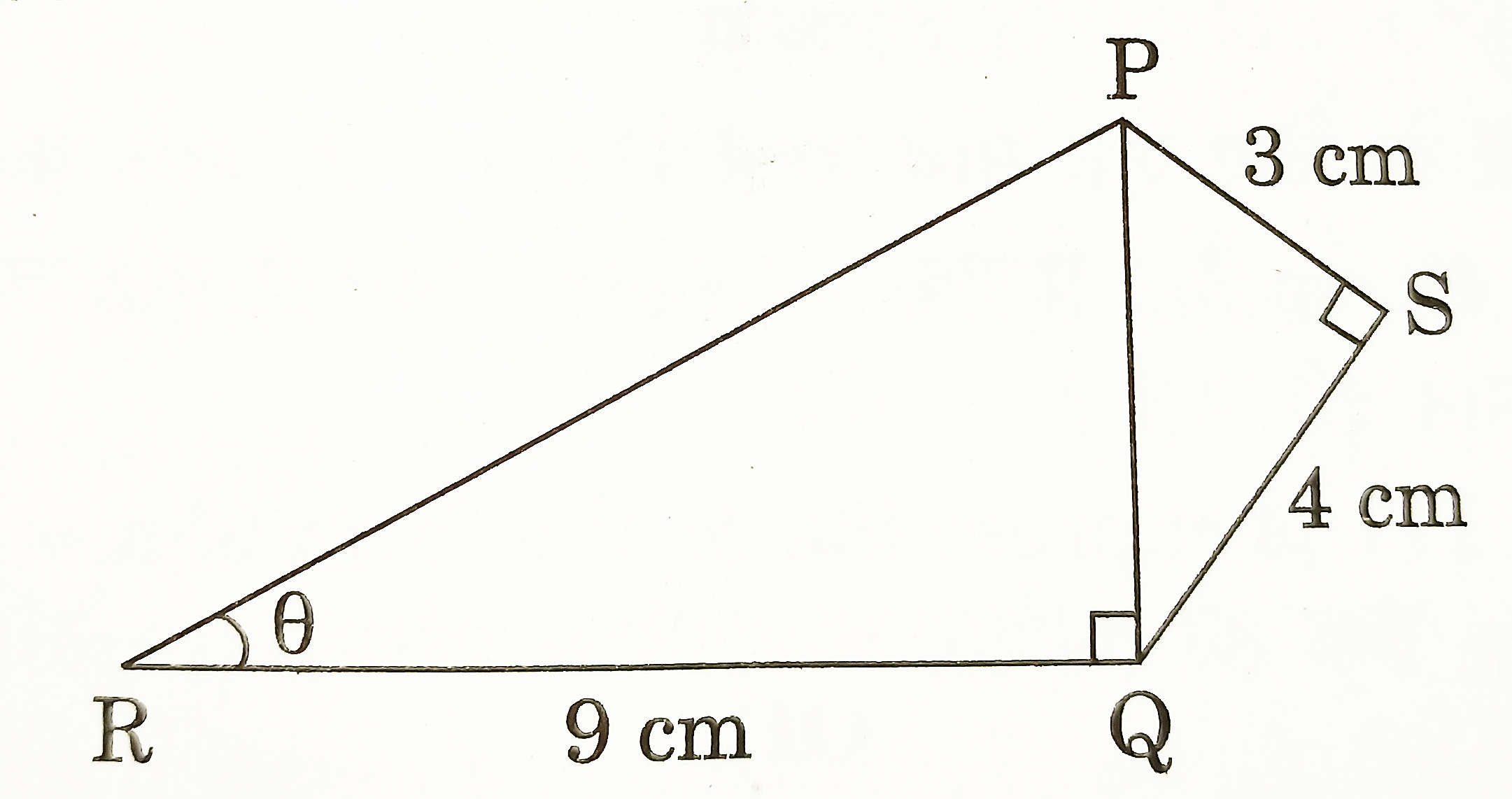# Class 10 – Introduction to Trigonometry – Previous years Questions

Introduction to Trigonometry – Previous years Questions

1. The value of θ for which cos(10° + θ) = sin 30° , is
1. 50°
2. 40°
3. 80°
4. 20° [CBSE 2020] [1 Mark]
2. The value of θ for which sin (44° + θ) = cos 30° , is
1. 46°
2. 60°
3. 16°
4. 90° [CBSE 2020] [1 Mark]
3. If tan A = 1, then 2 sin A cos A = ________. [CBSE 2020] [1 Mark]
4. What is the value of (cos2 67° – sin2 23°)? [CBSE 2018] [1 Mark]
5. In figure, PS = 3 cm, QS = 4 cm, ∠PRQ = θ, ∠PSQ = 90° , PQ RQ and RQ = 9 cm. Evaluate tan θ. [CBSE 2019] [1 Mark]6. If tan α = 5/12, find the value of sec α. [CBSE 2019] [1 Mark]
7. Evaluate:
[CBSE 2020] [2 Marks]
8. Evaluate:
[CBSE 2020] [2 Marks]
9. If 4 tan θ = 3, evaluate
. [CBSE 2018] [3 Marks]
10. If tan 2A = cot(A -18°), where 2A is an acute angle, find the value of A. [CBSE 2018] [3 Marks]
11. A, B and C are interior angles of a triangle ABC. Show that
1. If ∠A = 90°, then find the value of tan. [CBSE 2019] [3 Marks]
12. If tan (A+B) = 1 and tan (A-B) = 1/√3, 0° < A+B < 90°, A>B, then find the value of A and B. [CBSE 2019] [3 Marks]
13. Prove that :
[CBSE 2020] [3 Marks]
14. Prove that
. [CBSE 2018] [4 Marks]
15. If 1+sin2 θ = 3sin θ cos θ, then prove that tan θ = 1 or tan θ = 1/2. [CBSE 2019] [4 Marks]
16. Prove the following:
[CBSE 2019] [4 Marks]
17. Prove that:
[CBSE 2019] [4 Marks]